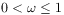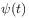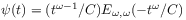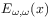• Fractional master equations and fractal time random walks
Sie sind hier: ICP » R. Hilfer » Publikationen

# Fractional master equations and fractal time random walks

###### Abstract.

Fractional master equations containing fractional time derivatives of orderare introduced on the basis of a recent classification of time generators in ergodic theory. It is shown that fractional master equations are contained as a special case within the traditional theory of continuous time random walks. The corresponding waiting time densityis obtained exactly aswhereis the generalized Mittag-Leffler function. This waiting time distribution is singular both in the long time as well as in the short time limit.

###### Key words and phrases:
continuous time random walks, fractional master equation
PACS: 05.40.+j,05.60.+w,02.50+s